# Principles and Progress in the Constructionof High-Speed Digital Computers

By ANDREW D. BOOTH and KATHLEEN H. V. BRITTEN
Birkbeck College, University of London, and
British Rubber Producers' Research Association,
Welwyn Garden City, Herts.

Quarterly Journal Mechanical and Applied Mathematics, Vol. II, Pt. 2 (1949)

Received 5 November 1947; revised 2 July 1948.

## Summary

The basic principles underlying the mathematical design of high-speed digital computers are discussed and the necessary components of such machines defined. Scale of notation, the form of the 'memory', the action of the control, and other practical details are considered, and a brief discussion is included of the exact arithmetic functions of which these machines must be capable.

This is followed by a description of current computer projects in America. Aiken's second relay computer at Harvard, the Bell relay machine, E.D.V.A.C., and the Princeton electronic computer are described briefly and an idea given of their state of completion in 1947.

## Introduction

It is the purpose of this paper to discuss the basic principles underlying the mathematical design of high-speed digital computers, and to follow this with an account of the progress which has been made, in the U.S.A. and at home, with the actual construction of such instruments.

## Digital versus analogue computers

At the outset it is perhaps worth noticing the essential difference between the two possible types of computing machine, digital and analogue.

An analogue machine is one which performs its functions by finding some mechanical, hydraulic, or electrical mechanism whose action in effect duplicates the mathematical processes required. A single example suffices; suppose that it is required to calculate the function a cos Ø. A simple mechanism for doing this is shown below.A cross-head OA is capable of rotation about O, a peg B is positioned on OA, so that OB = a. BC is a bar rigidly joined perpendicular to CP which is constrained to move in the line OCPX. If BC is maintained in contact with the peg B and OA is rotated through the required angle Ø, the displacement of P from its position when Ø = pi/2 is equal to a cos Ø. It is apparent that such a device will have severe limitations in accuracy and, for more complicated systems than that considered, an upper limit of 1 in 1,000 is expensive to realize, whilst 1 in 10,000 is on the extreme border of practicability. To paraphrase a remark of Professor Hartree, each decimal place multiplies the cost by a factor of about 10.

The most ambitious analogue computer constructed to date (and it now seems for all time) is the Bush-Caldwell differential analyser at the Centre of Analysis in the Massachusetts Institute of Technology; this achieves, on favourable occasions, the upper limit of accuracy quoted above and has a tape-feed device which enables a problem to be set into the machine from a standard commercial electrical typewriter. Electrical gear-boxes eliminate the laborious setting operation required in previous analysers, and the circuits are so arranged that all the integrators receive a fair share of the work of the machine. This feature is important in a machine whose maximum capacity exceeds that normally required on routine problems.

The subject of analogue computers will not be discussed further as the field is well known and is well covered in existing literature. A digital computer is defined to be any machine which takes its information in the form of actual numbers (i.e. assemblages of digits) and operates with it in this form throughout. It is at once apparent, from this, that only the ordinary operations of arithmetic are possible to such a machine, and that any continuous process must be replaced by a suitable finite difference or other numerical equivalent. The most common example of a digital computer is the ordinary desk Marchant, Monroe, or Brunsviga, and it is perhaps worth observing that such a machine, with a 6 x 6 decimal multiplication time of around 10 seconds, is faster by a factor of about 25 than a non-expert (i.e. non-professional computer) using pencil and paper methods. At these speeds it is just possible for the human operator to supply the machine with data and instructions at an adequate rate. With an increase in speed by even a factor of 10 this is no longer true and, if the machine is to be hand-controlled, no further improvement in performance is justified.

## The control of high-speed computers

These conditions lead naturally to the idea of a machine capable of controlling its own operations and exerting a certain amount of independent judgement. Before being more explicit it may be well to clear up a point which is often raised in this connexion. If a human operator has to 'programme', i.e. instruct the machine at some stage, does this not impose a fundamental limitation on the speed? The answer is in the negative, since most problems involve a large amount of repetition, and if the machine can exercise a little judgement it is sufficient to detail the process once and then tell the machine to repeat it, either a fixed number of times, or until some criterion is realized. As examples consider three typical problems:
1. Tabulation of f(x) for x = l,...,n;
2. Iterative evaluation of a1/2;
3. Multiplication of two matrices A . B.

In the first problem the detailed programme for the calculation of f (x) for one value of x would be written down. The next value of x would now be inserted into the scheme and the process repeated. The operation could be terminated by having the machine calculate y = (x-n) at each stage and then using a ' judgement' order of the type:

if y >= 0 perform operation sequence A;
but if y < 0 perform operation sequence B.

In this example sequence B would be that for calculating f(x), whereas A would signal the end of the calculation, since (x - n) < 0 until x = n. The process would therefore terminate automatically after the correct number of values of f(x) had been generated.

The word 'programme' as used in this context may require explanation. Before a problem is ready for solution in a computing machine it must first be broken up into processes which the machine is capable of performing. Thus, as mentioned above, when using a digital machine the continuous process of differentiation must be replaced by a finite difference formula. This translating of a problem in terms of the available functions of the machine is generally known as programming.

The second problem, the evaluation of a1/2 to a preassigned accuracy, is dealt with in the same fashion although the required number of iterations of the process

xn+l = (xn + a/xn)/2

may not be known ab initio. The decision to end the calculation is made by evaluating (xn+l - xn) at each stage and noticing that it is negative up to a certain point and zero afterwards (to a preassigned number of decimal places). The point at which the two successive approximations become equal is the required termination, and a ' judgement' order is adequate to determine it without human intervention.

The third example, matrix multiplication, is effected by a straight forward triple iteration and the same sort of procedure is adequate.

## The scale of notation

So far in this discussion of digital computers 'numbers' and 'digits' have been mentioned without definition, but one of the first problems confronting a designer of machines is the choice of a scale of notation for his numbers. The decimal, or scale of 10, notation, although hallowed by tradition, is by no means mandatory. With present electrical and electronic facilities it is an unfortunate fact that most simple devices favour not decimal but binary scale, and it is pertinent to consider what is in effect the best possible scale. To state the problem in mathematical terms: to record numbers up to a magnitude K, what scale of relationship minimizes the total number of digital positions required? To solve this, let n be the base and m the number of places (decimal or otherwise) required. The minimum value of D = nm subject to the condition nm = K has to be found. An easy application of the calculus gives the value

n = e.

The use of this transcendental number as a base is impracticable and 2, 3, or 4 are logically the next best choice. A numerical comparison is interesting; to record numbers up to 106 in decimal notation 60 digital positions are required, in binary or quaternary scales 40 digital positions, and in ternary scale 38 positions. The decimal notation is thus inefficient by a factor of about 1.5.

It is, of course, possible to adopt a 'binary decimal' scale, in which decimal digits are represented by their binary equivalents. Thus 8 would appear as (1000), 68 as (0110), (1000) etc. Using this representation, 48 positions would be required to represent numbers up to 106 and the scale is therefore inefficient by a factor of 1.2 compared with the binary notation. The choice between binary and ternary scale is dictated by the fact that the binary representation lends itself to easy application of 'relays' and 'flip-flops' the electronic equivalent of the relay.

One objection frequently raised against binary scale is the difficulty experienced by humans in the reading of large numbers of ones and zeros. As will be shown later, however, a properly designed machine can take its input in decimal form, convert to binary for its own operations, and then reconvert its output to decimal form. Another point must be mentioned in this connexion. Binary addition consists of three possible operations (1+1 = 10, 1+0 = 1, 0+0 = 0), whereas in decimal notation there are fifty-five different operations. Multiplication is correspondingly more complicated. The engineering problems to be solved in constructing a decimal machine are therefore much more formidable than those encountered when using binary scale. This objection applies equally to the 'binary-decimal' scale.

## Serial and parallel operation

The next point which arises in the design of a computer is the form of operation - serial or parallel. In the first type the digits of any number become available one at a time starting from the least significant, whilst, in the latter, all digits are simultaneously available. The decision as to which form of operation is to be used in any particular machine is one which can be made only when the form of the 'memory ' of the machine is known, and leads naturally to a discussion of the latter.

## General properties of the memory

It was mentioned earlier that human agencies are too slow to control machines which are much faster than those already in use. Some means of ordering the operations of the machine at speeds greatly in excess of neural reaction times is therefore required. This suggests that the machine should have a 'memory', and the characteristics of this will now be considered.

In the past, machines have been built in which the memory consists of two distinct parts, one for orders and the other for numbers. This is, as will be shown, an unsound arrangement, both from the viewpoint of logical arithmetic and from a much more elementary aspect. Two general classes of problem exist: those in which a small number of orders suffice for the handling of a large amount of numerical data, and those in which a large number of orders operate on a few numbers and produce a single number as a solution. If only a finite memory space is available, any idea of dividing it into two independent halves for orders and numbers respectively and exclusively is untenable on these grounds alone, and it will therefore be assumed that both orders and numbers are to be placed in the memory and a selection mechanism provided to pick out the correct material. This leads to the idea of a code.

## Basic components of a computer

If orders are to be stored in the same memory as numbers, they must be put into numerical form. This is relatively simple, but requires a careful consideration of the precise set of orders on which the machine is to operate. Before considering this, however, the operating components of the machine must be defined.

The main sequence of operations is as follows:

1. Control extracts order from memory.
2. Control decodes order and directs memory and arithmetic unit to execute it.
3. Arithmetic unit emits signal that its operation is complete.
4. Control locates next order in sequence and extracts it from the memory.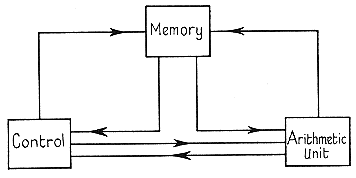Subsidiary sequences allow the control to jump to other orders not in sequence; these shifts may be either conditional or unconditional (vide infra).

The exact constitution of an order is of some importance; it is found that a satisfactory make up is:

'Perform operation A using data in memory position B.'

It is not necessary to specify the place of disposal of the result or the memory location of the next order. It will generally be convenient for the latter to be in sequence for the greater part of a calculation so that an automatic advance of the control (via a counter) performs this sequencing operation. For out-of-sequence orders special instructions are needed:

'Control execute order contained in memory position K and then proceed in sequence from this order',

which is the unconditional transfer referred to above; or

'If x < 0 execute order contained in memory position K and then proceed in sequence from this order',

which is the conditional transfer.

## The serial memory

More detailed description of the memory and arithmetic units depends almost entirely upon the details of the former. Serial memories suggested up to the present depend almost universally on the delay-line principle.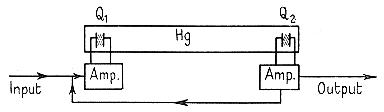A tube of liquid (usually mercury) has at its ends two quartz crystals Q1 and Q2. On applying an electric impulse to Q1, its piezo-electric property causes it to emit a mechanical pulse which is propagated down the mercury column as a compression wave. This compression wave affects a second quartz crystal Q2 which this time emits an electrical impulse; this impulse is led back to Q1 (after suitable amplification and reshaping) and the whole cycle repeats itself.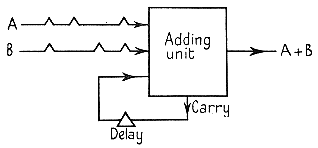The tubes in common use have a delay of about 1 millisecond so that if pulses are applied to Q1 at megacycle rate, up to 1,000 can be stored in the tube. A binary number now consists of an electrical impulse pattern of the type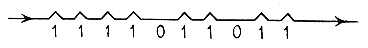The disadvantage of the scheme is of course that, on the average, 1/2 millisecond will elapse before any given number in the tube becomes available. To perform addition the two numbers are taken from different delay tubes and combined to form the sum, which is recorded in one of the original tubes. The addition of two binary digits may produce a carry; this has to be delayed by a time-interval delta and fed into the next pair of digits to become available, delta being the interval between pulses.

By a slightly more complicated arrangement, involving the storing of B in a short delay line, multiplication and division can be performed.

Professor F. C. Williams of Manchester has also developed a storage device. He uses a standard cathode-ray tube and stores data as charged areas on the screen, zero and one being represented by dots and dashes. Numbers are read off by scanning the rows of information, and digits are therefore obtained serially. Professor Williams has succeeded in storing 2048 digits in a 12 in. tube and in retaining information for what is effectively an indefinite period with no signs of deterioration.

## The parallel operation memory

Of the memories which have been or are being developed for parallel operation machines one of the most promising is the R.C.A. 'Selectron'. In essence this retains data by storage of charge on a dielectric medium. The mode of selection of the particular region for a given digit is interesting. A filament emits electrons in the direction of the dielectric medium which is shielded by two parallel grids of mutually perpendicular wires.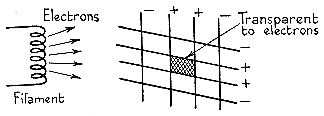Normally the grids are negative with respect to the filament; to select a particular region of the dielectric two adjacent wires in each grid are made positive; the region so defined becomes transparent to electrons, which can thus pass through and affect the dielectric storage medium.

## The arithmetic unit for parallel operation

In the parallel operation machine the digits become available at the same time, and different arrangements for addition, multiplication, and division have to be adopted. It is generally agreed that the arithmetic unit must consist of:
1. An accumulator; that is, a device which will add an incoming number to that already in it and store the result.
2. A register to store the multiplier in multiplication and the quotient in division.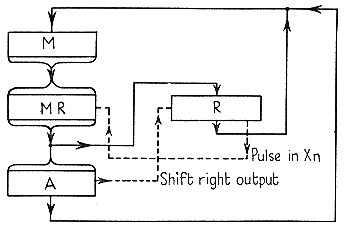In multiplication and division the multiplicand and the divisor are stored in a register associated with the output from the memory. The figure above gives a diagrammatic representation of the kind of arithmetic unit envisaged.

The register and accumulator have facilities which enable numbers contained therein to be shifted to the right or left. Interconnexions, indicated by full lines, are provided between the memory register (M.R.), the shifting register (R), and the accumulator (A) to enable numbers to be transferred from one unit to another. Numbers may also be transferred from the register and accumulator directly to the memory. The multiplication sequence (positive numbers only) is as follows:

If right-hand digit of the multiplier in the register R is unity add M.R. into A and shift contents of A one place to right, the right-hand digit of A going to the left-hand digit position of R whose contents also shift one place to the right (see dotted lines in figure above). The right-hand digit of R is lost and the next digit takes its place as the multiplier for the next cycle.

If the right-hand digit of R is zero the contents of A and R are simply shifted as before but without addition from M.R.

## The accumulator

It is interesting to examine the mode of operation of the accumulator. Just as in the serial type machine, the adding unit has two inputs together with a carry input from previous stages.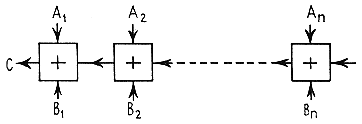This carry has been a source of speed limitation in many projects, since the speed of operation of the circuit has to be adjusted so that a carry (in the worst possible case) originating at An Bn can be propagated through the whole chain of adding units. This limitation is not, however, essential and the following circuit remedies the defect: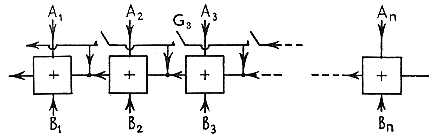Here, if an incoming carry pulse to any stage (say A3 B3) is going to produce a carry (i.e. if the result of the (A3 B3) addition is 1), the gate G3 is closed and the incoming pulse by-passes (A3 B3) and proceeds unhindered along the chain until it meets a stage which is not going to produce a further carry.

## The code

Enough has now been said to make clear the general mode of operation of the machine; it is proposed, however, before describing individual machines, to consider the question of the code in more detail. The following remarks will apply more to the parallel operation type of machine as it seems likely to replace the serial variety as being faster by at least an order of magnitude, and it is significant that Professor von Neumann, who pioneered the serial machine, has forsaken it in favour of a parallel operation type.

If the idea of programming all arithmetic out of the simplest operations is accepted, the list of orders will contain at least:

1. Left shift.
2. Right shift.
3. Control transfer.
4. Memory to accumulator.
5. Accumulator to memory.
6. Control transfer to order at memory location (x).

Out of this simple set all arithmetic operations can be generated, although greater detail will not be given here save to mention, as an example, that the nullity of a number can be sensed and a conditional control transfer generated from this simple set. This is simply done by successively separating (by suitable shifts) the digits, of the numbers whose nullity is required, and substituting into an order which sends the control to memory location (x); if the digit being substituted is different from zero, (x) is increased by unity and the order found at location (x+1) forms the start of a new sequence. In practice a more sophisticated code is adopted and as an example that of our own computer A.R.C. is given. The code for a serial type machine may be different in form and for details reference may be made to the report on A.C.E..

Although it has been shown how conditional transfer could be generated from logically simple operations, it is a great convenience to have this operation available in the code. It is interesting to consider its best formulation; two possibilities spring to mind:

1. Transfer if X < O.
2. Transfer if X = O.

One can be generated from the other, thus using form (1) consideration of -|X| gives (2), whereas given (2) a Consideration of |X| + X gives (1). From the engineering point of view (1) is more suited to a machine which represents negative numbers by their complements. The question of the representation of negatives is an important one. In some machines where magnitudes are restricted to |X| <= 1 it turns out that representation by complements is a valuable addition since it allows the use of the number -1.

## Code of A.R.C.

No.CodeSymbolDescription
10,0000Ti to MFill high-speed memory from input tape.
20,0001T to TiStart tape moving to position Ti and proceed with next order.
30,0010nTi to i+j to M1 to i+jRead material on nth tape between i and i+j into M.
40,0011M1 to i+j to TnPunch material from memory location i to i+j on to nth tape.
50,0100C to MShift control to order located at M(x).
60,0101Cc to MIf A > 0 shift control as in 5.
70,0110L(N)Shift contents of A and R, N places to left so that, e.g., A(0)-A(20), R(0)-R(20) to A(1)-A(20), R(0)-R(20), A(0).
80,0111R(N)Shift contents of A, N places to right so that, e.g., A(0)-A(20) to A(0), A(0)-A(I9).
90,1000M to cA
100,1001|M| to cA
110,1010-M to cA
120,1011-|M| to cA
130,1100M to A
140,1101|M| to A
150,1110-M to A
160,1111-|M| to A
171,0000M.R to cAClear A. Multiply M by R, place 1st 20 digits and sign in A after adding unity to 21st digit. Leave last 20 digits in R.
181,0001M.R to cA(N.R.)Perform multiplication without rounding off.
191,0010A ÷ M to cRDivide A by M, place quotient in R with last digit unity. Leave remainder in A.
201,0011M to cR
211,0100R to cA
221,0101R to M
231,0110A to M
241,0111AL to MSubstitute digits 1-8 of A in order located at M(x).
251,1000A to cR
261,1001ESignal completion of operation.

## Computer projects in America

We shall now give a short survey of current calculating machine projects in America. No description will be given of the E.N.I.A.C., as Hartree has provided this in his book Calculating Machines (C.U.P.). In any case, although this machine is of historic interest as the first large electronic calculator to operate successfully, it has been rapidly outmoded by recent developments, and cannot be regarded as typical of modern ideas on automatic computers.

## Aiken's relay computers

The A.S.C.C. (Automatic Sequence Controlled Calculator), built by Aiken at Harvard, also falls into this category. This relay controlled computer was completed in 1941 and has operated almost continuously since then to the present day. A very full description of this machine and its operation is given in A Manual of Operation for the A.S.C.C. published by Harvard.

Aiken has now completed a second, and larger, edition of this machine. The work of construction and wiring was completed last June (1947), testing was in progress, and the arithmetic unit had just been put into operating order.

The machine consists of the control and four arithmetic units. Input and output is via punched tape and results can be printed directly by the machine. It performs the elementary operations of addition, subtraction, multiplication and division, and has the discrimination order described above.

Aiken hopes to attain a multiplication time of the order of 1 sec., an improvement by a factor of 5 over the A.S.C.C., and a multiple arithmetic unit has been incorporated with the idea of speeding up the machine. This will, of course, greatly increase the complexity of programming, as in order to use the machine to best advantage all units must be working simultaneously. Indeed, Professor Aiken has mentioned as a major problem the difficulty of programming sufficient work to keep the machines continually busy.

Experience of programming for our own relay machine, A.R.C., has shown, however, that, using the code given above, quite complex problems (e.g. matrix multiplication) can be programmed in about 30 minutes, and it seems reasonable to suppose that most calculations could be dealt with in a matter of hours. It may be concluded from this that an increase in the speed and simplicity of the arithmetic unit is preferable to an increase in size.

The chief reason for the use of binary scale for automatic computers is the lack of a reasonably compact and economical form of decimal memory. An essential difference between Aiken's relay machines and other current projects is that they have only a very small memory and operate in decimal scale. Orders are taken in sequence from punched tape, and all results, intermediate or final, are recorded on tape. This increases the complexity of programming and reduces the speed of the machine, as reading from and recording on tape are comparatively slow operations.

The use of decimal scale, of course, eliminates the necessity for conversions at the beginning and end of every calculation and reduces the number of digits which must be carried, but it has the disadvantage that, from the engineering point of view, the operations of arithmetic are much more complicated in decimal than in binary scale.

In spite of these defects, however, both relay machines are fine examples of engineering, and Mark I has a most impressive record of continuous performance.

Aiken is also building an electronic computer, but development was not very far advanced last summer (1947). The main outlines of the machine had been decided upon, but the detailed circuits were not then designed. This machine is to have a high-speed memory, consisting of a drum with a number of magnetic tracks, each with its recording and pick-up head. Here again, decimal scale is to be used, decimal numbers being represented by a binary code.

## The Bell relay machine

The only other large relay computer in America has been constructed by Bell Telephones, and is now at the Aberdeen Proving Ground, Maryland. This machine is a general purpose calculator, built to the basic plan indicated earlier in this report. It uses modified binary scale, has tape input and output, and uses a code similar to that outlined above. It has been successfully used to solve various differential equations, and for the calculation of a table of the binomial function. The limitations of this machine lie in the size of the high-speed memory - twenty numbers, stored in relay banks - and the experience of those who have used it shows that this restriction in memory size both reduces the effective speed of the machine and increases the complexity of programming.

As is projected for other machines using binary scale, the Bell machine performs its own conversions to and from this scale. It has a certain degree of internal checking in that, when information is transferred from one part of the machine to another, the number of 1's occurring in the number before and after transfer are compared; if these are found to be different, the machine is automatically stopped. This is by no means a complete check, and indeed, no current project has yet found a really satisfactory solution to this problem. It has been proposed to run two identical machines, coupled in parallel, and so arranged that if the results produced at any stage are not identical, the calculation is stopped. This, however, is likely to be costly in practice, and, in any case, is not an absolute check since the comparing mechanism is liable to error.

## The E.D.V.A.C.

So far only 'parallel operation' machines have been described. Next comes the only overt project in America building a serial type computer, the E.D.V.A.C. (Electronic Discrete Variable Automatic Calculator) at the Moore School of Electrical Engineering, Philadelphia [Eckert and Mauchly's "Univac" is a commercial project and no details are available for publication]. This is to be an all-purpose, electronic computer using as memory, mercury delay-lines. Its counterparts in England are Wilkes's E.D.S.A.C. at Cambridge and the National Physical Laboratory's A.C.E.

In July (1947) the main body of E.D.V.A.C. was still under construction. A good deal of work has been done on delay-lines, and in particular on the problems arising from corrosion of the tanks by the mercury, and a satisfactory model has been adopted. The designs for the accumulator and control are complete, and a pilot model of the arithmetic unit was operating. This model, 'Shadrac' by name, performs addition, subtraction, multiplication and division, but no controls were built in and numbers had to be inserted by hand. 'Shadrac' was built for experimental purposes only and has a capacity for twenty digit binary numbers, the delay lines being about 18 in. long. The final machine will work to forty binary digits and on the basis of experience gained with 'Shadrac', it is hoped to achieve a multiplication time of 1 millisecond.

A typical order will consist of four parts:

• Specification of the locations of the two numbers to be operated upon.
• Operation (+, -, etc.).
• Location to which result is to be sent.

Some coded examples are given in a report on E.D.V.A.C. (not published) and these indicate that coding for the machine is a comparatively quick and easy process. Orders and data will be punched on teletype tape, transferred to magnetized wire, and thence to the machine. This rather complicated process is necessary since direct tape input would be far too slow for the machine. The same method is to be adopted for the project next to be described, that of von Neumann at the Institute for Advanced Study, Princeton.

## The Princeton electronic computer

This is probably the most ambitious project at present in existence, as it is hoped to attain a multiplication time of less than 100µ sec. The machine is to be a parallel type electronic computer using binary scale. It differs from all those discussed so far in the size of its memory; this is hoped to be about 4,000, forty digit binary numbers to be stored in the 'selectrons' briefly described above. The machine will follow the general plan described earlier in this report, and will consist of the memory, accumulator, shifting register, control, and the input and output units.

The input is to be via teletype tape and magnetized wire, and the machine will be provided with a library of these wires containing tabulated functions and codes for routine calculations.

The code is again similar to that described above, and each order will consist of two parts:

1. The operation to be performed.
2. The memory location of the number to be operated upon.

Conversions to and from binary scale will be done by the machine, decimal numbers being inserted in binary coded form. The large memory capacity, and simple code, will make the coding of problems for this machine comparatively rapid and easy.

At the end of last summer a shifting register and accumulator were in operation, and the magnetic input and output was complete. The accumulator had an addition time of 2µ sec., but it was hoped to improve on this; the control circuits necessary to make multiplication and division possible had not, at that time, been completed.

A great deal of work has been done on the best and most economical medium for the magnetic input and wire has been chosen as providing minimum bulk with good recording capacity. To avoid undue bulk, small gauge wire will be used, and difficulty was experienced at first because the tensions set up when starting and stopping caused breakages. This problem has been solved by mounting two drums on a common shaft and winding the wire from one to the other via the reading and recording heads. The effective radii of these drums will, of course, vary slightly with the amount of wire on them, but the consequent tensions in the wire are eliminated by the use of a differential gear and a small servo-motor.

Another problem which has to be considered in this connexion is that of reading from the wire while accelerating. It is possible to lose digits in this process, and this is to be avoided by having a set of marker pulses between each 'word'. A counting device records the number of digits read out between each marker pulse, and if any are missed, the word is rejected. It is hoped, in the final machine, to record 100 digits to the inch and to run the wire at 50 ft. per sec., giving an input speed of 60,000 digits per sec.

Progress on the memory has, so far, been slow. Originally it was to consist of forty 'selectrons', each containing 4,000 digits. At the end of the summer (1947), however, no working model of the selectron had been produced, and it seems that, when this is available, it may contain, at first, only 256 digits. While this memory is adequate for a relay machine, it will probably be quite insufficient for the much faster electronic computer, and it may be necessary to develop an alternative form of high-speed memory consisting of, for example, magnetized drums.

Forrester, of the Massachusetts Institute of Technology, is engaged on an almost identical project to that of von Neumann. Development is slightly behind that at Princeton, but the only essential difference is in the proposed form of the high-speed memory. Forrester hopes to use some form of electrostatic storage, presumably of the general type suggested by Williams.

Although the United States is comparatively rich in computers and computer projects, it is interesting to note that all are sponsored, in part at any rate, by the Government, and that all, with the exception of the Princeton machine and Forrester's computer, are eventually to be moved to the Aberdeen proving ground or to the U.S. Navy Proving Ground at Dahlgren, Virginia.

## References

1. Francis Murray, The Theory of Mathematical Machines. King's Crown Press, New York.
2. For example, REICH, The Theory and Application of Electron Tubes, McGraw-Hill, 1945.
3. A.C.E. Report, National Physical Laboratory, 1948.

Converted for the Web by Bob Mackay